# Water Resource Management Model¶

Public authority must decide how much water to release from a reservoir so as to maximize benefits derived from agricultural and recreational uses.

• States
• s reservoiur level at beginning of summer
• Actions
• x quantity of water released for irrigation
• Parameters
• a0,a1 -- producer benefit function parameters
• b0,b1 -- recreational user benefit function parameters
• $\mu$ -- mean rainfall
• $\sigma$ -- rainfall volatility
• $\delta$ -- discount factor
In :
import numpy as np
import matplotlib.pyplot as plt
from compecon import BasisChebyshev, DPmodel, DPoptions, qnwlogn, demo
import seaborn as sns
import pandas as pd


### Model parameters¶

In :
a0, a1, b0, b1 = 1, -2, 2, -3
μ, σ, δ = 1.0, 0.2, 0.9


The deterministic steady-state values for this model are

In :
xstar = 1.0  # action
sstar = 1.0 + (a0*(1-δ)/b0)**(1/b1)  # stock


### State space¶

The state variable is s="Reservoir Level", which we restrict to $s\in[2, 8]$.

Here, we represent it with a Chebyshev basis, with $n=15$ nodes.

In :
n, smin, smax = 15, 2, 8
basis = BasisChebyshev(n, smin, smax, labels=['Reservoir'])


### Continuous state shock distribution¶

In :
m = 3  #number of rainfall shocks
e, w = qnwlogn(m, np.log(μ)-σ**2/2,σ**2) # rainfall shocks and proabilities


### Action space¶

The choice variable x="Irrigation" must be nonnegative.

In :
def bounds(s, i=None, j=None):
return np.zeros_like(s), 1.0*s


### Reward function¶

The reward function is

In :
def reward(s, x, i=None, j=None):
sx = s-x
u = (a0/(1+a1))*x**(1+a1) + (b0/(1+b1))*sx**(1+b1)
ux = a0*x**a1 - b0*sx**b1
uxx = a0*a1*x**(a1-1) + b0*b1*sx**(b1-1)
return u, ux, uxx


### State transition function¶

Next period, reservoir level wealth will be equal to current level minus irrigation plus random rainfall:

In :
def transition(s, x, i=None, j=None, in_=None, e=None):
g = s - x + e
gx = -np.ones_like(s)
gxx = np.zeros_like(s)
return g, gx, gxx


### Model structure¶

The value of wealth $s$ satisfies the Bellman equation \begin{equation*} V(s) = \max_x\left\{\left(\frac{a_0}{1+a_1}\right)x^{1+a1} + \left(\frac{b_0}{1+b_1}\right)(s-x)^{1+b1}+ \delta V(s-x+e) \right\} \end{equation*}

To solve and simulate this model,use the CompEcon class DPmodel

In :
water_model = DPmodel(basis, reward, transition, bounds,e=e,w=w,
x=['Irrigation'],
discount=δ )


## Solving the model¶

### Compute Linear-Quadratic Approximation at Collocation Nodes¶

The DPmodel.lqapprox solves the linear-quadratic approximation, in this case arround the steady-state. It returns a LQmodel which works similar to the DPmodel object. We compute the solution at the basis nodes to use it as initial guess for the Newton's methods.

In :
water_lq = water_model.lqapprox(sstar,xstar).solution(basis.nodes)


Solving the growth model by collocation. We take the values for the Value and Policy functions at the basis nodes obtained from the linear-quadratic approximation as initial guess values for the Newton's method.

In :
S = water_model.solve(v=water_lq['value'], x=water_lq['Irrigation'])

Solving infinite-horizon model collocation equation by Newton's method
iter change       time
------------------------------
0       7.1e-01    0.0156
1       1.2e-01    0.0378
2       1.2e-02    0.0534
3       1.3e-04    0.0690
4       1.9e-08    0.0846
5       1.5e-14    0.0846
Elapsed Time =    0.08 Seconds

Out:
Reservoir value resid Irrigation
Reservoir
2.000000 2.000000 -14.086357 8.445569e-07 0.628126
2.040268 2.040268 -13.985981 -6.417778e-07 0.638659
2.080537 2.080537 -13.888843 -7.616363e-07 0.649069
2.120805 2.120805 -13.794753 -3.379168e-07 0.659357
2.161074 2.161074 -13.703538 1.731421e-07 0.669527

DPmodel.solve returns a pandas DataFrame with the following data:

We are also interested in the shadow price of wealth (the first derivative of the value function).

In :
S['shadow price'] = water_model.Value(S['Reservoir'],1)

Out:
Reservoir value resid Irrigation shadow price
Reservoir
2.000000 2.000000 -14.086357 8.445569e-07 0.628126 2.534519
2.040268 2.040268 -13.985981 -6.417778e-07 0.638659 2.451652
2.080537 2.080537 -13.888843 -7.616363e-07 0.649069 2.373665
2.120805 2.120805 -13.794753 -3.379168e-07 0.659357 2.300174
2.161074 2.161074 -13.703538 1.731421e-07 0.669527 2.230828

## Plotting the results¶

### Optimal Policy¶

In :
fig1 = demo.figure('Optimal Irrigation Policy', 'Reservoir Level', 'Irrigation')
plt.plot(S['Irrigation'])
demo.annotate(sstar, xstar,f'$s^*$ = {sstar:.2f}\n$x^*$ = {xstar:.2f}','bo', (10, -6),ms=10,fs=11)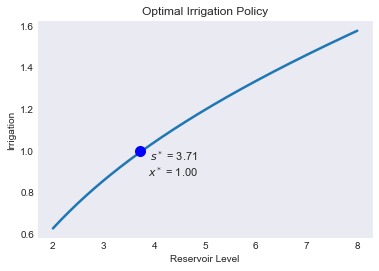### Value Function¶

In :
fig2 = demo.figure('Value Function', 'Reservoir Level', 'Value')
plt.plot(S['value'])

Out:
[<matplotlib.lines.Line2D at 0x2941207d160>]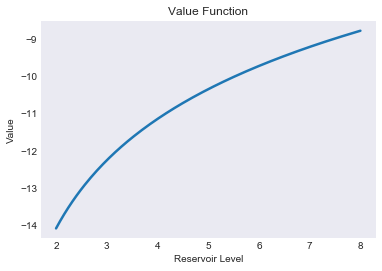In :
fig3 = demo.figure('Shadow Price Function', 'Reservoir Level', 'Shadow Price')

Out:
[<matplotlib.lines.Line2D at 0x29412298240>]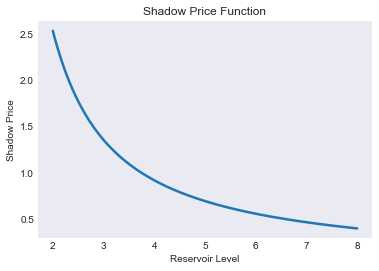### Chebychev Collocation Residual¶

In :
fig4 = demo.figure('Bellman Equation Residual', 'Reservoir Level', 'Residual')
plt.hlines(0,smin,smax,'k',linestyles='--')
plt.plot(S[['resid']])
plt.ticklabel_format(style='sci', axis='y', scilimits=(-1,1))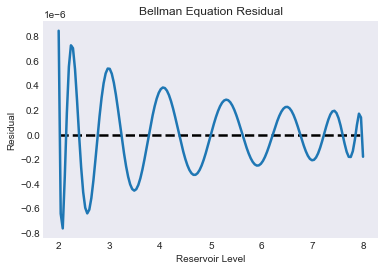## Simulating the model¶

We simulate 21 periods of the model starting from $s=s_{\min}$

In :
T = 31
nrep = 100_000
data = water_model.simulate(T, np.tile(smin,nrep))


### Simulated State and Policy Paths¶

In :
subdata = data[data['_rep'].isin(range(3))]
opts = dict(spec='r*', offset=(0, -15), fs=11, ha='right')

In :
fig6 = demo.figure('Simulated and Expected Reservoir Level','Year', 'Reservoir Level',[0, T + 0.5])
plt.plot(data[['time','Reservoir']].groupby('time').mean())
plt.plot(subdata.pivot('time','_rep','Reservoir'),lw=1)
demo.annotate(T, sstar, f'steady-state reservoir\n = {sstar:.2f}', **opts)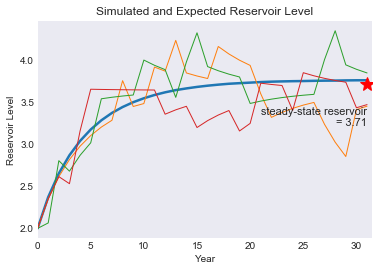In :
fig7 = demo.figure('Simulated and Expected Irrigation','Year', 'Irrigation',[0, T + 0.5])
plt.plot(data[['time','Irrigation']].groupby('time').mean())
plt.plot(subdata.pivot('time','_rep','Irrigation'),lw=1)
demo.annotate(T, xstar, f'steady-state irrigation\n = {xstar:.2f}', **opts)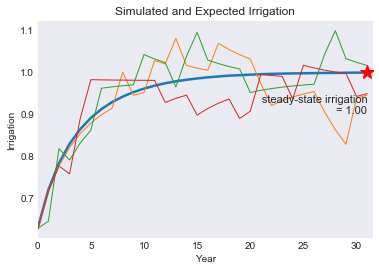### Ergodic Wealth Distribution¶

In :
subdata = data[data['time']==T][['Reservoir','Irrigation']]
'Ergodic Means': subdata.mean(),
'Ergodic Standard Deviations': subdata.std()}).T
stats

Out:
Reservoir Irrigation
fig8 = demo.figure('Ergodic Reservoir and Irrigation Distribution','Wealth','Probability')

<matplotlib.axes._subplots.AxesSubplot at 0x29423eaf5c0>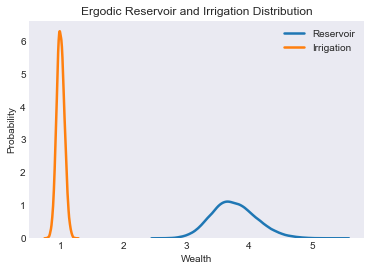#demo.savefig([fig1,fig2,fig3,fig4,fig5,fig6,fig7,fig8])